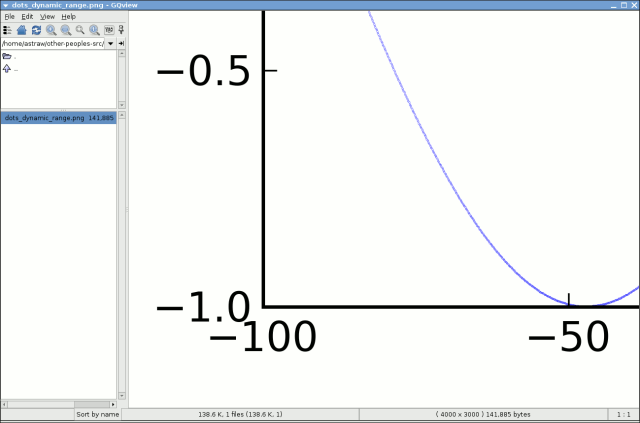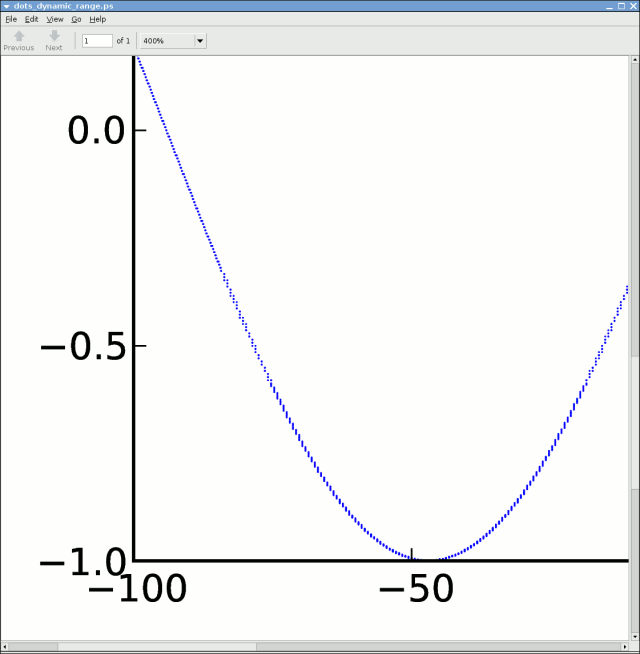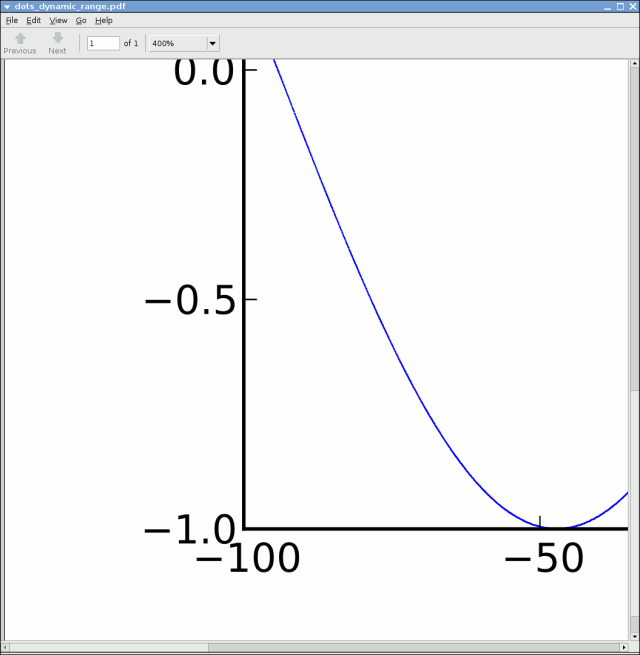# bug: ps backend postion rounding?

Hi,

When I attempt to plot small-scale features with the PS backend, it
seems that there's a granularity at which things cannot be further
specified. I cannot remember encoutering this before, although I can't
be sure it's not an old phenomenon. Anyhow, the following script
illustrates the issue. With the PS backend, zooming in on the output
shows the points spread significantly away from their locations that
would be expected.

import matplotlib
matplotlib.use('PS')
import pylab
import numpy

x=numpy.linspace(-100,100,2000)
x+=0.001*numpy.random.rand(len(x))

y=numpy.sin(x/30.0)
y+=0.001*numpy.random.rand(len(x))

pylab.plot(x,y,'.',ms=0.1)
pylab.savefig('dots_dynamic_range')

With the Agg and PDF backends, this does not occur. I'm attaching a few
example images which hopefully make the issue clear. (These have all
seen a little bit of processing to reduce the file sizes.)Hi Andrew,

···

On Friday 20 June 2008 19:28:20 Andrew Straw wrote:

When I attempt to plot small-scale features with the PS backend, it
seems that there's a granularity at which things cannot be further
specified. I cannot remember encoutering this before, although I can't
be sure it's not an old phenomenon. Anyhow, the following script
illustrates the issue. With the PS backend, zooming in on the output
shows the points spread significantly away from their locations that
would be expected.

import matplotlib
matplotlib.use('PS')
import pylab
import numpy

x=numpy.linspace(-100,100,2000)
x+=0.001*numpy.random.rand(len(x))

y=numpy.sin(x/30.0)
y+=0.001*numpy.random.rand(len(x))

pylab.plot(x,y,'.',ms=0.1)
pylab.savefig('dots_dynamic_range')

With the Agg and PDF backends, this does not occur. I'm attaching a few
example images which hopefully make the issue clear. (These have all
seen a little bit of processing to reduce the file sizes.)

I see this also. I checked the text contents of the ps file, and the precision
after the decimal place is lost. This is surprising behavior to me:

In : '%1.3g %1.3g'% (429.120463939, 155.230815676)
Out: '429 155'

I changed it back to "%g %g", like it is in the v91 branch.

Darren